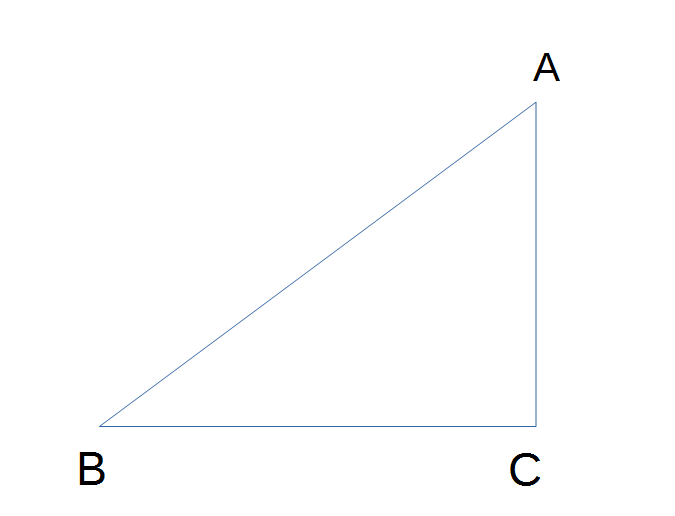# Sine and CosineSuppose there is a right triangle. Sine is the height divided by the hypotenuse, and cosine is the base divided by the hypotenuse.

Sine is often notated as sin, and cosine as cos.

sin:
cos:

sin0 = 0
sin30 = ½
sin90 = 1

cos0 = 1
cos30 = ½√3
cos90 = 0

Updated:

Tags:   math